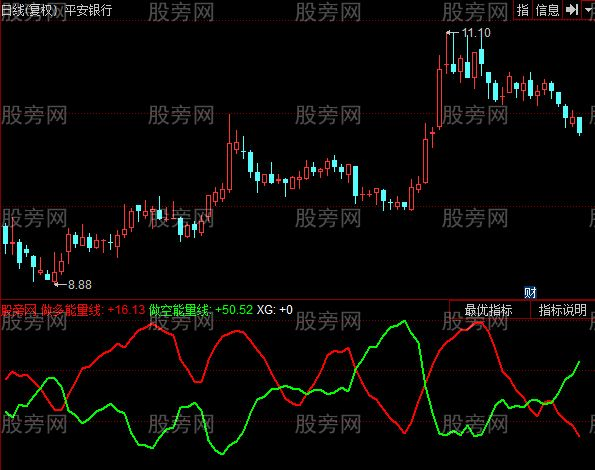# 同花顺板块轮动指标公式

Var3:=100-3*SMA((CLOSE-LLV(LOW,75))/(HHV(HIGH,75)-LLV(LOW,75))*100,20,1)+2*SMA(SMA((CLOSE-LLV(LOW,75))/(HHV(HIGH,75)-LLV(LOW,75))*100,20,1),15,1);
Var4:=100-3*SMA((OPEN-LLV(LOW,75))/(HHV(HIGH,75)-LLV(LOW,75))*100,20,1)+2*SMA(SMA((OPEN-LLV(LOW,75))/(HHV(HIGH,75)-LLV(LOW,75))*100,20,1),15,1);
Var5:=Var3-Var4;
Var27:=Var3<REF(Var4,1) AND VOL>REF(VOL,1) AND CLOSE>REF(CLOSE,1);DRAWTEXT(Var27 AND COUNT(Var27,30)=1,50,'资金'),colorred;
VAR3:=100-3*SMA((CLOSE-LLV(LOW,75))/(HHV(HIGH,75)-LLV(LOW,75))*100,20,1)+2*SMA(SMA((CLOSE-LLV(LOW,75))/(HHV(HIGH,75)-LLV(LOW,75))*100,20,1),15,1);
VAR4:=100-3*SMA((OPEN-LLV(LOW,75))/(HHV(HIGH,75)-LLV(LOW,75))*100,20,1)+2*SMA(SMA((OPEN-LLV(LOW,75))/(HHV(HIGH,75)-LLV(LOW,75))*100,20,1),15,1);
VAR27:=VAR3<REF(VAR4,1) AND VOL>REF(VOL,1) AND CLOSE>REF(CLOSE,1);
XG:VAR27 AND COUNT(VAR27,30)=1,colorwhite;